# SRMJEEE Maths Mock Test - 2

## 35 Questions MCQ Test SRMJEEE Subject Wise & Full Length Mock Tests | SRMJEEE Maths Mock Test - 2

Description
Attempt SRMJEEE Maths Mock Test - 2 | 35 questions in 50 minutes | Mock test for JEE preparation | Free important questions MCQ to study SRMJEEE Subject Wise & Full Length Mock Tests for JEE Exam | Download free PDF with solutions
QUESTION: 1

Solution:
QUESTION: 2

Solution:
QUESTION: 3

### Let f(x) = [cos x + sin x], 0 < x < 2π where [x] denotes the greatest integer less than or equal to x. The number of points of discontinuity of f(x) is

Solution: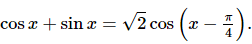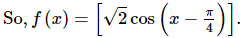We know that [x] is discontinuous at integral values of x.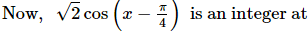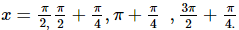QUESTION: 4

Equation of the circle having diameters 2x - 3y = 5 and 3x - 4y = 7 and radius 8 is

Solution: The intersection of the diagonals i.e. (1,-1) is the centre of the circle with radius 8. put in the formula
(x-1)^2+(y+1)^2=8^2
x2+y2-2x+2y-62=0
QUESTION: 5

Coordinates of two points are A(1,0) and B(-1,0) and Q is a point which satisfies AQ-BQ = + 1. The locus of the point Q is

Solution: Option B is correct.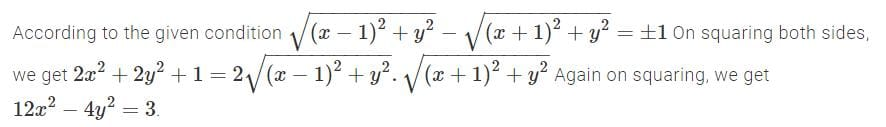QUESTION: 6

The term independent of x in the expansion of ((2x) - (3/x))6 is

Solution:
QUESTION: 7

The equation of tangent at point (1,2) to the parabola y2=4x is

Solution:
QUESTION: 8

The number of solutions of the equation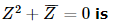Solution:
QUESTION: 9

The area bounded by the curve y=sinx, y=0, x=0 and x=(π/2) is

Solution:
QUESTION: 10

The family of curves, in which the subtangent at any point to any curve is double the abscissa, is given by

Solution:
QUESTION: 11

If the matrix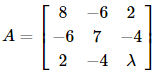is singular one, then λ is

Solution:
QUESTION: 12

∫x sec2x dx=

Solution:
QUESTION: 13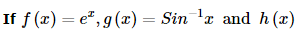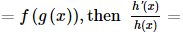Solution:
QUESTION: 14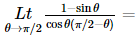Solution:
QUESTION: 15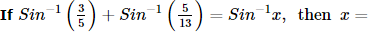Solution:
QUESTION: 16

If A and B are 2 x 2 matrices then (A+B)2=

Solution:
QUESTION: 17

The ratio of the altitude of the cone of greatest volume which can be inscribed in a given sphere to the diameter of the sphere is

Solution:
QUESTION: 18

The mean of 3, y, 4, x, 10, is 5, then y =

Solution:
QUESTION: 19

The inverse of matrix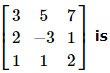Solution:
QUESTION: 20

In a certain distribution, the following results were obtained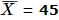, Median = 48, Coefficient of skewness = -0.3.

What is the value of standard deviation?

Solution:
QUESTION: 21

The angle between lines y2sin2θ-xysin2θ+x2(cos2θ-1) = 1 is

Solution:
QUESTION: 22

Three identical dice are rolled. The probability that the same number will appear on each of them is

Solution:
QUESTION: 23

If the sides of a triangle are proportional to the cosines of the opposite angles then the triangle is

Solution:
QUESTION: 24

If the difference between the corresponding roots of x2 + ax + b = 0 and x2 + bx + a = 0 is same and a ≠ b, then

Solution:
QUESTION: 25

If A.M. between two numbers is 5 and their G.M. is 4, then their H.M. is

Solution:

If x, y and z respectively represent AM, GM and HM between two numbers a and b, then
y2 = xz
Here x = 5, y = 4
then 16 = 5 x z
z = 16/5

QUESTION: 26

The number of 4-digit even numbers that can be formed using 0, 1, 2, 3, 4, 5, 6 without repetition is

Solution:

Each even number must have 0, 2, 4 or 6 in is units place
Here total number of digits = 7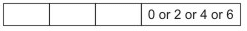When 0 occurs at units place there is no restriction on other places and when 2 or 4 occurs at units place there is restriction on thousands' place as 0 can not be put at thousands' place
Case I When 0 occurs at units place: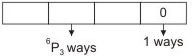∴ The number of numbers formed in this case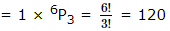Case II. When 0 does not occur at units place: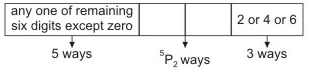The units' place can be filled up by any one of the three digits 2, 4 and 6 in 3 ways
∴ The number of numbers formed in this case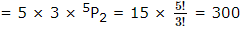∴ The required number = 120 + 300 = 420

QUESTION: 27

A condition for a function y = f (x) to have an inverse is that it should be

Solution:
QUESTION: 28

The derivative of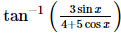is given by

Solution:
QUESTION: 29

A line passing through point A(-5,-4) meet other three lines x + 3y + 2 = 0, 2x + y + 4 = 0 and x - y - 5 = 0 at B, C and D respectively. If (15/AB )2 + (10/AC)2 = (6/AD)2, then the equation of line is

Solution:

Equation of any line through A(-5, -4) is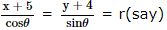then the coordinates of any point on this line at a distance r from A are
(r cosθ - 5, r sinθ - 4)
If AB = r1, AC = r2, AD = r3
then (r1 cosθ - 5, r1 sinθ - 4) lies on x + 3y + 2 = 0
⇒ r1 cosθ - 5 + 3 (r1 sinθ - 4) + 2 = 0
⇒ r1 (cosθ + 3 sinθ) = 15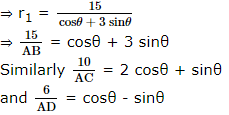Therefore according to the given condition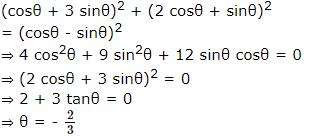Hence the required equation of the line is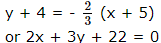QUESTION: 30

X follows a binomial distribution with parameters n = 6 and p. If 4 P (X = 4) = P (X = 2), then p =

Solution:
QUESTION: 31

The curve y-exy+x=0 has a vertical tangent at the point

Solution:
QUESTION: 32

The equation 3sin2x + 10cosx - 6 = 0 is satisfied if

Solution:
QUESTION: 33

The minimum value of sin x-cos x is

Solution:
QUESTION: 34

A tetrahedron has vertices at O (0,0,0), A(1,2,1), B(2,1,3) and C(-1,1,2), then the angle between the faces OAB and ABC will be

Solution:
QUESTION: 35

If a=2i+j-k, b=i+2j+k and c=i-j+2k then a.(bxc)=

Solution:Use Code STAYHOME200 and get INR 200 additional OFF Use Coupon Code